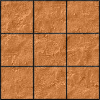#### You may also like### Paving the Way

A man paved a square courtyard and then decided that it was too small. He took up the tiles, bought 100 more and used them to pave another square courtyard. How many tiles did he use altogether?### Square Areas

Can you work out the area of the inner square and give an explanation of how you did it?### Chess

What would be the smallest number of moves needed to move a Knight from a chess set from one corner to the opposite corner of a 99 by 99 square board?

# Opposite Vertices

##### Age 11 to 14 Challenge Level:

Here is how Emily from Beeston Rylands Junior School started on the problem.

Damini from Newstead Wood School For Girls discovered that not all diagonal lines that join two grid points make a square:

You find the centre of your diagonal line and go from there away from the line in a straight line (at right angles) until you find the right point. Then join the point to one end of the diagonal line and you have a side! How easy was that. Not all diagonal lines that join two grid points make a square. If you take a piece of square dotty paper and join up any two dots it will be hard to find the square, as there might not even be a square.

I agree with the fact that a straight line can be the side of many different rhombuses but to me I see no real way of determining how many rhombuses go to one line.

I also agree that the diagonal of one rhombus can have the same diagonal line as infinitely many other rhombuses as if you take one line you can keep editing the size of the rhombus without actually changing the length of the first diagonal.

Alexander from Wilson's School suggested a strategy for finding the number of possible rhombuses that could be made when he is given a side:

To find how many different rhombuses can be found with a single line that is between two dots, you need to choose one end of the line. You then look at "one side" of that point, and see how many different ways the line can be "twisted". You then multiply it by four because the same number of "twisted" lines can be found on the "other side" of that point, and on the "two sides" of the other end of the line. The answer to this operation determines how many rhombuses can be made from one line on a dotted piece of paper.
(Editor's comment: I think that Alexander has double counted here; each of the "twisted lines" found at one end will make rhombuses with each of the "twisted lines" found at the other end of the line.)

Niharika from Leicester High School for Girls explained that if the vector $\pmatrix{a\cr b}$ represents the diagonal line, the square will have vertices at grid points if a+b (and therefore a-b) is even.

She also suggested a strategy for finding the number of rhombuses that could be drawn if one of the sides is given. Her solution is here.

Niharika also contributed a solution to  Vector Journeys, which uses vector notation so solve a related problem.

We also received good responses from the Maths Galaxy Explorers from North Walsham Junior School.